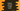# How to convert a tuple to set in Python## How to convert a tuple to set in Python:

Tuple and set, both are inbuilt data types in python. Tuple is similar to list, but it is immutable and ordered. Again, set is used to hold unique items in Python. It is mutable.

Python provides a way to convert a tuple to set easily. In this post, we will learn how to do that with examples.

## set() function:

The set() function is used to create a set in Python. It takes one iterable and create a set from it:

``set([iterable])``

We can pass a tuple to this function and it will convert it to a set.

### Example program:

Let’s take a look at the below method:

``````given_tuple = ('hello', 'world', 123, '!!', 123, 'world')

new_set = set(given_tuple)

print(f"tuple: {given_tuple}")
print(f"set: {new_set}")``````

Here,

• given_tuple is the tuple. We need to use () to create a tuple in Python.
• new_set is the set created from this tuple, given_tuple.
• The last two lines are printing the values of the tuple and set.

If you run it, it will print the below output:

``````tuple: ('hello', 'world', 123, '!!', 123, 'world')
set: {'hello', 123, 'world', '!!'}``````

As you can see here, it converted the tuple to a set. Since we can’t have duplicate items in a set, all duplicates from the tuple are removed in the set.

### Example 2:

Let’s take another example:

``````given_tuple = (1, 2, 3, 4, 5, 6, 7, 8, 9, 10, 9, 8, 7, 6, 5, 4, 3, 2, 1)

new_set = set(given_tuple)

print(f"tuple: {given_tuple}")
print(f"set: {new_set}")``````

It will print:

``````tuple: (1, 2, 3, 4, 5, 6, 7, 8, 9, 10, 9, 8, 7, 6, 5, 4, 3, 2, 1)
set: {1, 2, 3, 4, 5, 6, 7, 8, 9, 10}``````